MPA-HOMEPAGE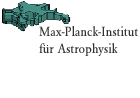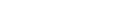Quickfinder FAQ job vacancies   about the institute research groups local phone list weekly seminars preprints library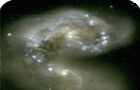Topology of the UniverseMPA Homepage > Scientific Research > Research Groups Topology of the UniverseGo to:

-Introduction
-Simulation Parameters
-Data
-References
-Credit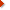Data Visualization (images & movies from Numerical Simulation projects)VIRGO MembersVIRGO Links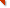Main U.K. SitePublic Announcement
of the VIRGO data
releaseIntroduction

Galaxy bias and the cosmic variance: We use two large CDM simulations to study the statistical properties of biased "galaxy" distribution and to estimate the sample variance due to the choice of the observer position, so-called cosmic variance. For each simulations we employ two empirical bias schemes such that the lowest order statistical property (two-point correlation function) of the "galaxies" closely resembles that of observed galaxies. We then use the Minkowski functionals to measure higher-order statistics.Simulation parameters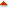Please seeVery Large Simulations.Figure 1 Distribution of the model biased "galaxies"

 Bias t1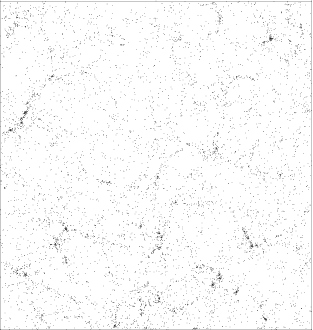Bias L1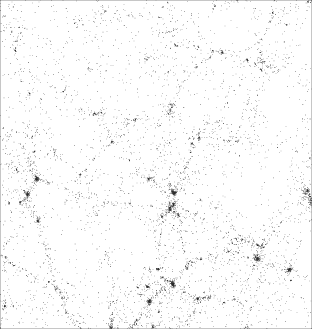Bias t2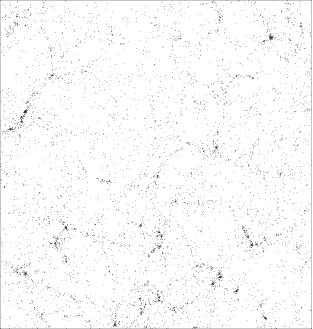Bias L2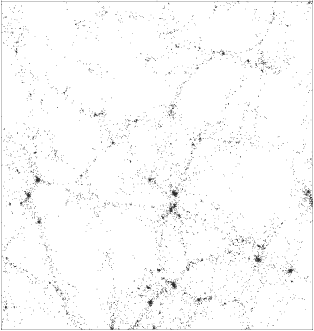Figure 2 Two-point correlation functions of the biased "galaxies"

Shown below are the computed two-point correlation functions for our 4 biased "galaxy" samples. The observed correlation function (by APM galaxy survey) is plotted as open squares. Although all of our samples have similar values for this statistics, there is a significant difference in their higher-order statistics, see Figure 3 below. It is interesting that the 'galaxy' distributions look very different as shown in Figure 1.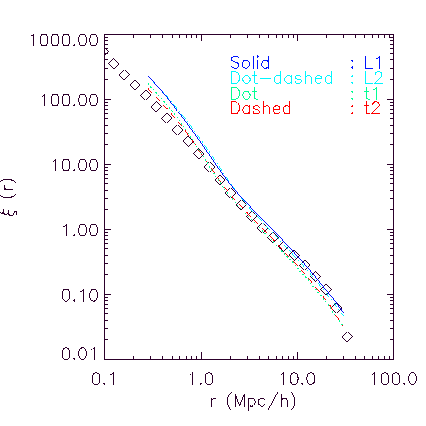Figure 3 The Minkowski functional (v1) measured for the biased "galaxies"

The quantity v1 for the 'galaxy' distributions are plotted against the "ball radii", bias L1 (solid lines) and bias L2(dotted line).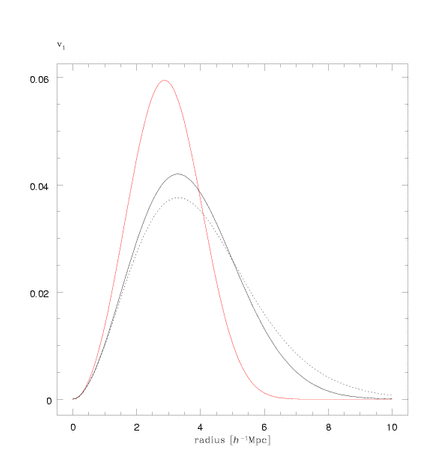Schmalzing, Yoshida, Diaferio, in preparation.Here is an example of proper credit for these Virgo data.
"The simulations in this paper were carried out by the Virgo Supercomputing Consortium using computers based at Computing Centre of the Max-Planck Society in Garching and at the Edinburgh Parallel Computing Centre. The data are publicly available at www.mpa-garching.mpg.de/galform/virgo/vls/resim.shtml"Comments to: N. Yoshida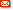naoki@mpa-garching.mpg.de